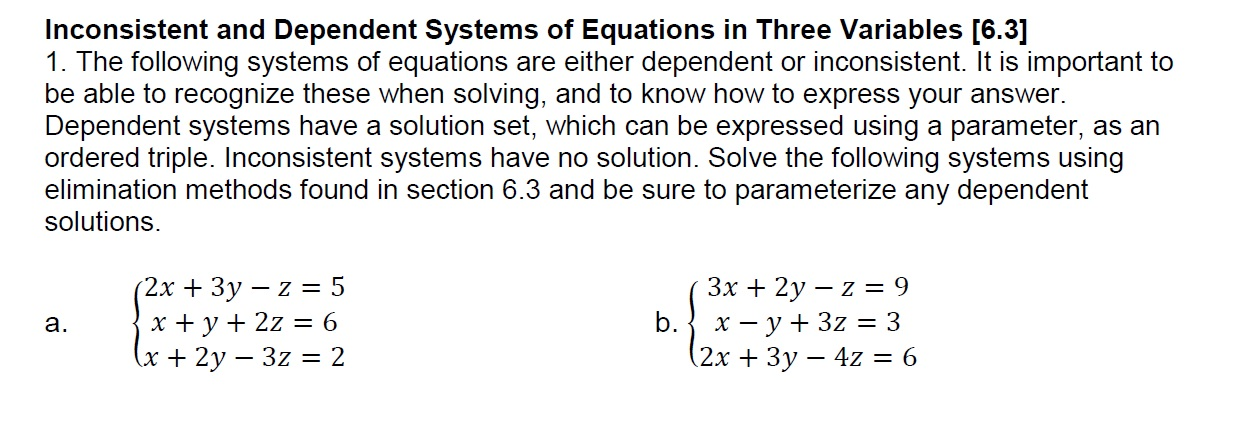# Inconsistent and Dependent Systems of Equations in Three Variables [6.3]1. The following systems of equations are either dependent or inconsistent. It is important tobe able to recognize these when solving, and to know how to express your answerDependent systems have a solution set, which can be expressed using a parameter, as anordered triple. Inconsistent systems have no solution. Solve the following systems usingelimination methods found in section 6.3 and be sure to parameterize any dependentsolutions.2x 3y - z 5a.2x +3y - 4z-6

Question
22 viewshelp_outlineImage TranscriptioncloseInconsistent and Dependent Systems of Equations in Three Variables [6.3] 1. The following systems of equations are either dependent or inconsistent. It is important to be able to recognize these when solving, and to know how to express your answer Dependent systems have a solution set, which can be expressed using a parameter, as an ordered triple. Inconsistent systems have no solution. Solve the following systems using elimination methods found in section 6.3 and be sure to parameterize any dependent solutions. 2x 3y - z 5 a. 2x +3y - 4z-6 fullscreen
check_circle

Step 1

By eliminating x from first and second equation, we get y-5z=-7  and by eliminating x from second and third equation , we get  y-5z=-4.

These equations make no sence , because frome these two equations , we have -7=-4, that is not possible.

So , these equations are inconsistent.

Step 2

By eliminating x from first and second and then from second and third, we get same equation y-2z=0. So. the system of equation is cons...

### Want to see the full answer?

See Solution

#### Want to see this answer and more?

Solutions are written by subject experts who are available 24/7. Questions are typically answered within 1 hour.*

See Solution
*Response times may vary by subject and question.
Tagged in

### Equations and In-equations